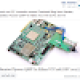# Seminar Report on PID Controller

Introduction to PID controller

Proportional-Integral-Derivative controller or PID controller are widely used in the industrial control applications. These are preferred due to its robust characteristics against changes at the system model. Unlike other controllers, PID controller can adjust process inputs based on the history and rate of change of the error signal, which gives more accurate and stable control.  There are many methods such as first order, second order and tuning methods. But, all general methods can be applied for control design of PID controller.

Brief into working of PID controller

The PID controller compares the  output value (y) with the reference set point (r) value . Then the error signal is generated. The error signal is then processed and the control signal is calculated such that the output reaches desired value. There are three parameters the Proportional, the Integral and Derivative values which are mainly involved in the calculation procedure.

The Proportional value is used to determine the reaction to the current error; the Integral value is used for the reaction based on the sum of recent errors, and the Derivative value for determining the reaction based on the rate at which the error has been changing. If these three parameters are not chooses correctly, then this leads to the instability.

To avoid these tuning methods must be applied. Manual tuning method is done if the system has to be online and this does not require any math’s, Ziegler Nichols method is the proven method and used when the system has be online.  Software tools method is the constituent tuning method, the system can be online or offline. Cohen-coon method is the offline method and can be used only for first order processes.

1.tonmay says:
2.ahmed says: